Platform: All Platforms Applies to: COMSOL Multiphysics®, , RF Module, MEMS Module, Plasma Module, Acoustics Module, Wave Optics Module, Semiconductor Module Versions: All versions

## Problem Description

Should I use the RMS, the peak, or the instantaneous value to specify sources in models being solved in the frequency domain, such as electromagnetic or acoustic models? How can I specify the phase angle?

## Solution

The COMSOL Multiphysics formulation uses complex fields when solving time-harmonic problems in electromagnetics, acoustics, and structural mechanics, or any other physics where the Frequency Domain study type is being used. For example, when solving electromagnetic problems, one is often solving for the electric field: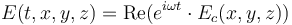where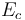is the complex electric field that you are entering,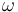is the angular frequency,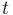is time, and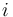is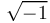.

Whenever you specify a source field you can input a complex number (a phasor) such as `Ec` where `abs(Ec)` is the peak value, and `real(Ec)` gives you the instantaneous value at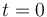.

This applies for any time-harmonic input. So, to define for instance an external current density, a magnetic field, an acoustic pressure, or an electric potential, with an RMS value of 1 and a phase angle of 30°, enter the expression:

`sqrt(2)*exp(i*30[deg])`

or, alternatively:

`sqrt(2)*(cos(30[deg])+i*sin(30[deg]))`